# GRE Subject Test: Physics : Quantum Mechanics and Atomic Physics

## Example Questions

### Example Question #1 : Quantum Mechanics And Atomic Physics

Which of the following wavelengths could be used to measure the position of an electron with the greatest accuracy?

Infared

Gamma-rays

Red light

X-ray

Gamma-rays

Explanation:

The Heisenberg Uncertainty principle states that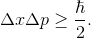Therefore, the smallest wavelength will have the lowest uncertainty in the position. Thus, we must pick the regime of options given which has the lowest wavelength. That is gamma-ray.

### Example Question #1 : Quantum Mechanics And Atomic Physics

What wavelength will result in the most accurate measurement of the momentum of an electron?

Infrared

Red light

Gamma-ray

X-ray

Explanation:

The Heisenberg Uncertainty Principle states that:Since the uncertainty in the position is inversely proportional to the uncertainty in the momentum, we need to pick the longest wavelength. Of the options listed, radio has the longest wavelength.

### Example Question #1 : Quantum Mechanics And Atomic Physics

If a ground state particle is in a one-dimension square well, where is the probability of finding the particle equal to zero?the way in the box.

At the boundary.

In the middle.

None of thesethe way in the box.

At the boundary.

Explanation:

This is a fundamental concept question. For a ground state particle, the only place where the probability is equal to zero is at the boundary because the particle cannot be found there. He has to be in the box (not unlike a cat).

### Example Question #2 : Quantum Mechanics And Atomic Physics

What is the energy of the photon emitted when a Hydrogen atom makes a transition from the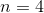to the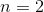atomic energy level?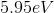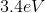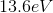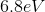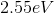Explanation: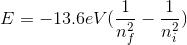, whererefers to the final and initial energy levels, respectively.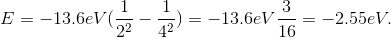However, since the question asked what is the energy of the photon, it is the absolute value of the energy calculated above because of conservation of energy. The photon carries away the energy lost by the atom.

### Example Question #1 : Quantum Mechanics And Atomic Physics

What color will be emitted in the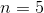totransition in the Hydrogen Balmer series?

Yellow

Blue

Red

Green

Violet

Violet

Explanation: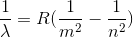.

Here,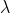is the wavelength,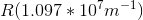is the Rydberg constant,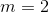for the Balmer series, andrefers to the upper atomic level (this isin this case). Now, we just need to plug everything in, and solve for wavelength.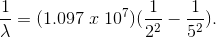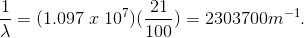By inverting,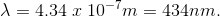This is in the violet regime of the visible spectrum.

### All GRE Subject Test: Physics Resources